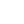# Defintion of Divide and Conquer

Divide the problem into smaller problem, use the result of the small problems to form the result of the bigger problem.

# Merge Sort

Divide the elements into two parts, recursively divide each parts until two elements are remained, merge each part together.

Each level operation is n Total running time = each level big O * number of level Number of level = 2 log2 n = n * 2 log2 n = n log2 n Running time: `O(n log n)`

## Sample code written in C

``````#include <stdio.h>
#include <stdlib.h>

// merge the element at [start]...[end] into temp array
// and copy back to the array size
void merge(int *array, int start, int mid, int end, int *temp){
int i = start;
int j = mid + 1;
int counter = 0;

while ( (i <= mid) && (j <= end) ){
if (array[i] < array[j]){
temp[counter++] = array[i];
i++;
} else {
temp[counter++] = array[j];
j++;
}
}

// Only either of the while loop will be executed
while ( i <= mid ){
temp[counter++] = array[i];
i++;
}
while ( j <= end){
temp[counter++] = array[j];
j++;
}

// copy back to the original array
for (i = start; i <= end ; i++){
array[i] = temp[i - start];
}

}

void divide(int *array, int start, int end, int *temp){
// no more divide for less than 2 element in the array
int mid = (start + end) / 2;
if (end - start < 2){
merge(array, start, mid, end, temp);
}
else {
divide(array, start, mid, temp);
divide(array, mid + 1, end, temp);
merge(array, start, mid, end, temp);
}

/* To you can also write the codes as
int mid = (start + end) / 2;
if (end > start){
divide(array, start, mid, temp);
divide(array, mid + 1, end, temp);
merge(array, start, mid, end, temp);
} */

/* Or if (end <= start) return; */

}

// non recursive version
// array: array to be sorted
// start: start index
// end : end index
void mergeSort(int *array, int start, int end){
int *temp = (int *)malloc(sizeof(int) * (end - start + 1));
divide(array, start, end, temp);
free(temp);
}

int main(void){
int nums [] = {2, 5, 0, 9, 1};
mergeSort(nums, 0, 4);

for (int i = 0 ; i< 5; i++){
printf("%d, ", nums[i]);
}

return 0;
}``````

## Counting inversion

Counting inversion is one of the application of merge sort. In the "merge process", it can be used to "count the inversion".

``````  if (array contains only one element)
return 0

Divide array into A & B
a = Sort-and-Count(A)
b = Sort-and-Count(B)
c = Merge-and-Count(A, B)

return a + b + c;``````

# Cloest Pair

It can be done by dividing the panel into left and right panel and finding the closest pair recursively in each part. To find the closes pair perhaps connecting from left panel to right panel we need to test 2 * beta distance which beta is defined as `min(closest_distance(left), closest_distance(right))`

``````[Left][Right]
[Left<-beta->][<-beta->Right]``````

People find that in order to find the closest pair of an particular point in beta area, they just need to test its 11-th consecutive point in y coordinate let yg be the elements in the beta element

``````for (int i = 0; i < yg.length; i++)
for (int j = 1; j <= 11; j++)
if (distance(yg[i], yg[i+j] < beta)
beta = distance(yg[i], yg[i+j])``````